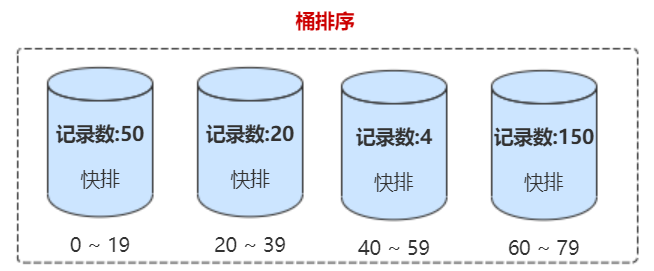# Java 排序算法 - 线性排序：桶排序和基数排序

## 1. 桶排序

### 1.1 桶排序原理分析### 1.2 桶排序使用场景

• 数据能划分成 m 个桶，并且桶之间有着天然的大小顺序。这样每个桶内的数据都排序完之后，桶与桶之间的数据不需要再进行排序。
• 数据在各个桶之间的分布是比较均匀的。如果数据经过桶的划分之后，有些桶里的数据非常多，有些非常少，很不平均，那桶内数据排序的时间复杂度就不是常量级了。在极端情况下，如果数据都被划分到一个桶里，那就退化为 O(nlogn) 的排序算法了。

## 2. 计数排序

### 2.1 计数排序原理分析

int[] arr = {2, 5, 3, 0, 2, 3, 0, 3};  // 原始数组
int[] tmp = {0, 0, 0, 0, 0, 0, 0, 0};  // 临时数组，存放排序后的数组
0  1  2  3  4  5  6  7
int[] c1 = {2, 0, 2, 3, 0, 1}          // 统计每个元素有多少个
int[] c2 = {2, 2, 4, 7, 7, 8}          // 统计累加元素有多少个
0  1  2  3  4  5


for (int i = n - 1; i >= 0; --i) {
int k = c[arr[i]] - 1;   // 1. 根据累加个数，计算元素在有序元素中的位置 k
r[k] = arr[i];           // 2. 将元素放到临时的有序数组中 tmp
c[arr[i]]--;             // 3. 如果有元素值 value 相同的，则放到 k-1 的位置
}


public void sort(Integer[] arr) {
if (arr.length <= 1) return;

int n = arr.length;
// 1. 查找数组中数据的范围
int max = arr;
for (int i = 1; i < n; ++i) {
if (max < arr[i]) {
max = arr[i];
}
}

// 2. 申请一个计数数组c，下标大小[0,max]
int[] c = new int[max + 1];
for (int i = 0; i <= max; ++i) {
c[i] = 0;
}

// 3. 计算每个元素的个数，放入c中
for (int i = 0; i < n; ++i) {
c[arr[i]]++;
}
// 4. 依次累加
for (int i = 1; i <= max; ++i) {
c[i] = c[i - 1] + c[i];
}

// 临时数组r，存储排序之后的结果
int[] r = new int[n];

// 5. 计数排序核心步骤：依次将数组arr放到有序数组r中
for (int i = n - 1; i >= 0; --i) {
int index = c[arr[i]] - 1;
r[index] = arr[i];
c[arr[i]]--;
}

// 6. 将结果拷贝给a数组
for (int i = 0; i < n; ++i) {
arr[i] = r[i];
}
}


## 3. 基数排序

### 3.1 基数排序原理分析

15172354984
13972354985


### 3.2 基数排序使用场景

1. 需要分割出独立的"位"来比较，而且位之间可以进行比较。
2. 每一位的数据范围不能太大，要可以用线性排序算法来排序，否则，基数排序的时间复杂度就无法做到 O(n)。
3. 如果排序的元素位数不一样，位数不够的可以在后面补位。

1. 排序动画演示：http://www.jsons.cn/sort/

posted on 2020-03-06 15:27  binarylei  阅读(184)  评论(0编辑  收藏

• 随笔 - 425
• 文章 - 0
• 评论 - 15
• 引用 - 0